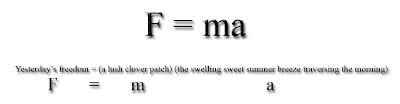## Sunday, August 12, 2007

Unlike a simple mathematical structure as in the “Similar Triangles Poem”, the “Paradigm Poem” is a mathematical poetry technique that borrows its structure from an existing equation of scientific or cultural significance. The “Paradigm Poem has many sub-categories which are as numerous as there are categories for applied mathematics. Examples that we could consider would be: “Physics Paradigm Poem”, “Chemistry Paradigm Poem”, “Business Accounting Paradigm Poem”, “ Psychophysical Paradigm Poem” etc.
If we think in terms of metaphor using the cognitive scientific language of George Lakoff then we would classify the language of the variables “inside the equation structure” as the ‘target domain’ and the context or traditional meaning of the equation as the “source domain’
Let me show an example of a “Physics Paradigm Poem” using Newton’s second law. For this example I am going to use an excerpt from my essay on “Polyaesthetics and Mathematical Poetry” Journal of Mathematics and the Arts, Volume 1, Issue 1 March 2007 , pages 35 - 40 Publisher: Taylor & Francis Informa Ltd Registered in England and Wales Registered Number: 1072954

“My personal view is that almost all mathematic applications rely on using equations with the intent similar to simile. The variables in the equation are compared explicitly with the result for uses in denotation. For example, in the case of an application of
Newton’s second law F = ma, or Force equals Mass times Acceleration, we are comparing the variables m (Mass) and a (Acceleration) explicitly to F (Force).
I can now make a mathematical poem based on the latter example by expressing the Force of ‘yesterday’s freedom’ as being equal to ‘a lush clover patch’
multiplied by ‘the swelling sweet summer breeze traversing the morning’. We can then put this in the form of a mathematical equation as; Yesterday’s freedom = (a lush clover patch) (the swelling sweet summer breeze traversing the morning). In other words, I set the Force to ‘Yesterday’s freedom’, the mass to ‘a lush clover patch’, and I accelerated the mass by ‘the swelling sweet summer breeze traversing the morning’. All of these
phrases relate back to the original equation from physics F = ma. I want to emphasize that I was very careful when I made my choice for acceleration so that the phrase is evocative of the mathematical description of acceleration as defined by physics. Acceleration is the
change in velocity of an object per unit of elapsed time during that acceleration. Here, the change in velocity is implied by ‘swelling’ and the change in time is implied by ‘traversing’.”In the above example we are using the words “Yesterday’s freedom; a lush clover patch; the swelling sweet summer breeze traversing the morning” as the variables of the equations which supply us with the target domain and then we are using the equation from physics F = ma, its scientific meaning and historical significance as the source domain.

The bottom line concerning the ‘paradigm poem’ is that we borrow an equation from the past which inherently contains historical significance and serves as an paradigm or mathematical model that seems almost “a vessel” to carry the mathematical poem. The paradigm poem always borrows an existing mathematical structure to serve as a source domain in our metaphor.Anonymous said...

Based on some of your poems, I decided to make one of my own based on a graph theory book I was reading...it is certainly not as subtle as your work, but I think that it came out fairly interesting. As background,the Euler-Schlaefli Equation for Dimension three is V - E + F - C = 0, where V=number of vertices of a polyhedra, E=number of edges of the polyhedra, F=number of faces of the polyhedra, and C=number of cells of the polyhedra (a cell is the number of areas space is divided into by the polyhedra).

Point-to-point, each vertex strives –
V lonely souls,
cries the Euler-Schlaefli.
Elements of dimension null
walk logic’s edge…

-

and reach ascension;
E outreaching arms,
cries the Euler-Schlaefli,
Dance along in dimension one,
look face-to-face…

+

at the midnight sun.
F all-seeing eyes,
cries the Euler-Schlaefli.
Mirror ‘pon mirror in dimension two,
reflecting their bigotry…

-

isolating you
in one of C cells.
cries the Euler-Schlaefli –
a battle cry of prejudice
echoing through dimension three,
where you always have more choices
but where you’ll never be as free.

=

Void.
Vacuum.
An empty world.
And even here, in barren land,
"Order!"
,cries the Euler-Schlaefli
and order came
and structure was
so space
is

Kaz Maslanka said...

I LIKE IT!

Thanks!
Kaz